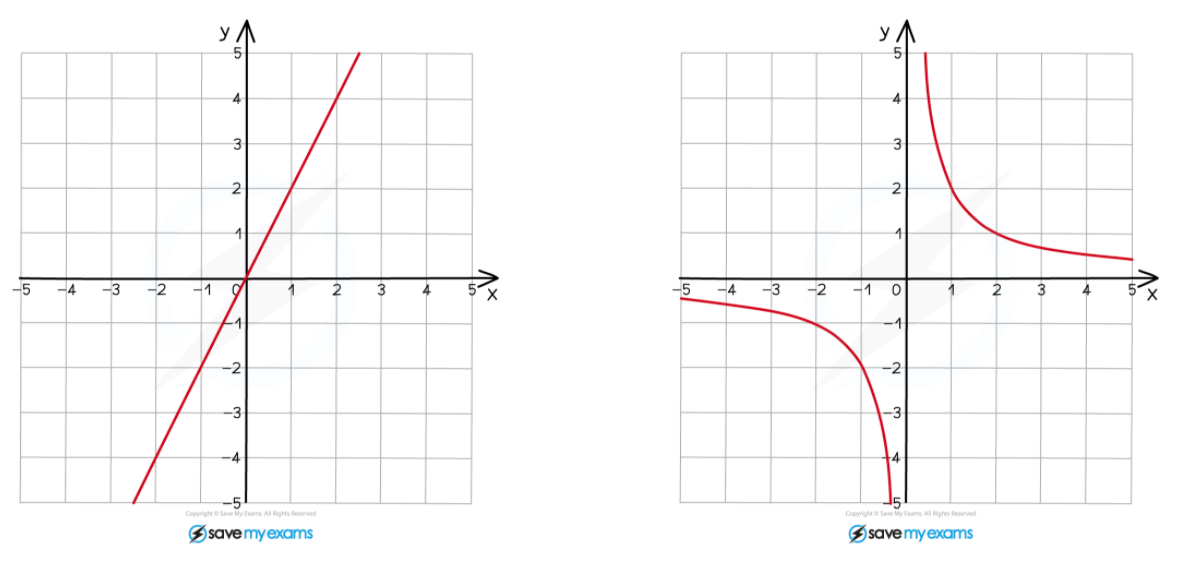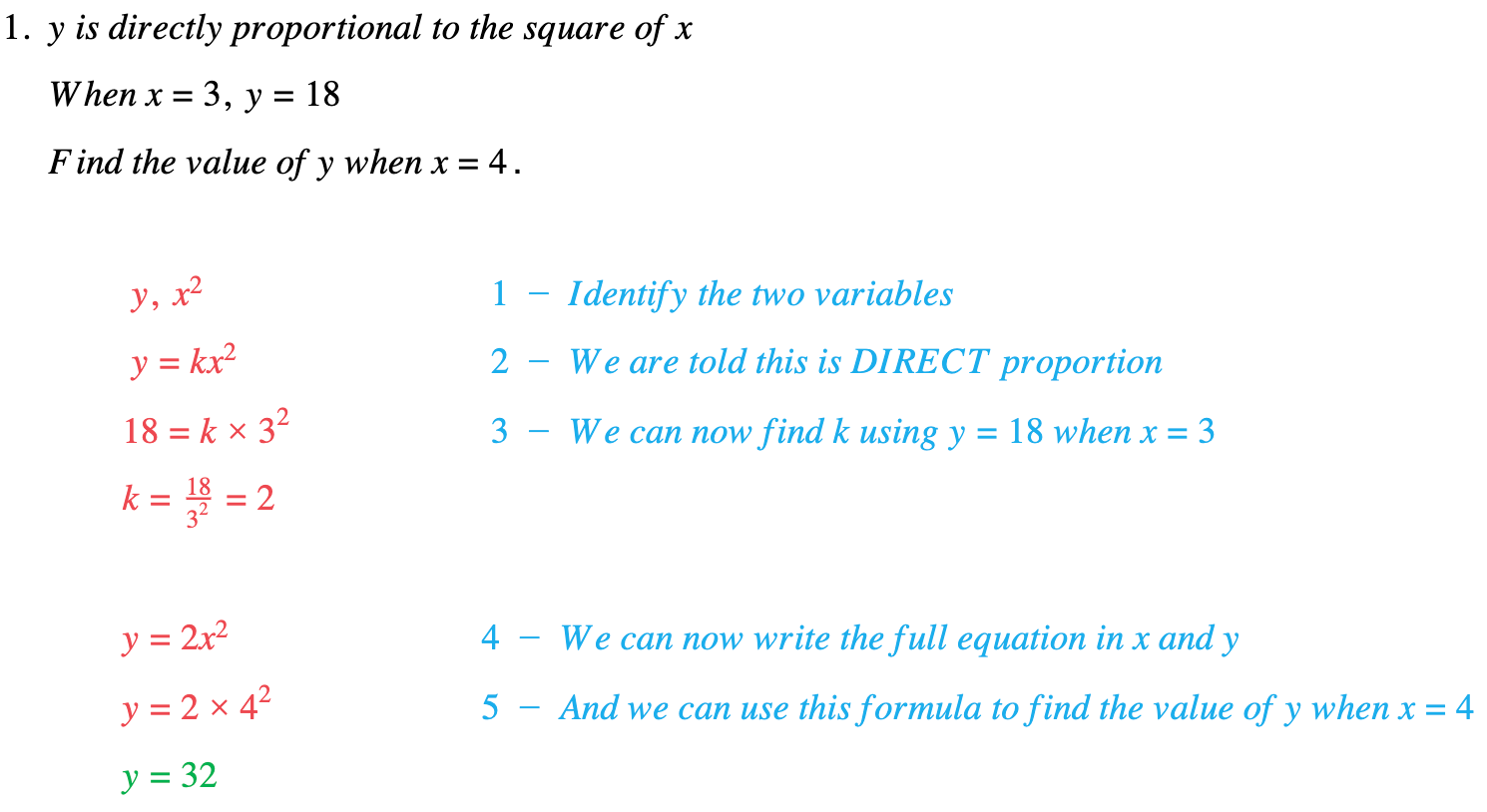# Edexcel IGCSE Maths 复习笔记 2.12.1 Direct & Inverse Proportion

Edexcel IGCSE Maths 复习笔记 2.12.1 Direct & Inverse Proportion

#### What is proportion?

• Proportion is a way of talking about how two variables are related to each other
• Direct proportion: as one variable goes up the other goes up by the same factor

eg. If one variable is multiplied by 3, so is the other

• Inverse proportion: as one variable goes up the other goes down by the same factor

eg. If one variable is multiplied by 3, the other is divided by 3

You should know what the graphs look like:#### How do we deal with proportion questions?

1. Identify the two VARIABLES (called A and B below)
2. Choose TYPE of proportion:

A is DIRECTLY proportional to B use formula A = kBA is INVERSELY proportional to B use formula A = k÷B

3. FIND k using the values given in the question
4. Write FORMULA for A in terms of B (using your value of k)
5. USE formula to find the required quantity

#### Exam Tip

Even if the question doesn’t ask for a formula (Step 4. above) it is always worth working one out and using it in all but the simplest cases.

#### Worked Example